• 用c 语言分别实现采用首次适应算法和最佳适应算法的动态分区分配过程alloc()和回收过程free(),其中，空闲分区通过空闲分区链来管理；在进行内存分配时，系统优先使用空闲区低端的空间。。。。 假设初始状态下，可用...
• 课程设计报告 课程设计题目循环首次适应的动态分区分配算法模拟 专 业计算机科学与技术 班 级10204102 姓 名谱 学 号: 10204102 指导教师: 高小辉 2013年 1 目 录 一循环首次适应算法 3 1. 概述 3 2需求分析3 二实验...
• 操作系统实验，使用首次适应算法和最佳适应算法对作业进行分配内存和回收内存
• 为把一个新作业装入内存，需按照一定的分配方法，从空闲分区表分出一分区分配给作业，最佳适应算法是将将空闲分区以容量从小到大的顺序排列，使用数组来存放空闲分区，使用冒泡法排序，算法优先使用内存中较小的空闲...
• 编写程序模拟实现内存的动态分区法存储管理内存空闲区使用自由链管理,采用最坏适应算法从自由链中寻找空闲区进行分配,内存回收时要与相邻空闲区的合并 初始状态信息:假定系统的内存共640K,初始状态为操作系统本身...
• 最佳适应算法源程序代码 #include <iostream.h> #include <iomanip.h> //全局变量 float minsize=5; int count1=0; int count2=0; #define M 10 //假定系统允许的空闲区表最大为m #define N 10 //假定系统允许的最大...
• 首次适应算法（First Fit）、 最佳适应算法（Best Fit）、 最坏适应算法（Worst Fit）【又称“最大适应算法”（Largest Fit）】、 临近适应算法（Next Fit）

学习地址（哔哩哔哩网站——王道论坛）：https://www.bilibili.com/video/BV1YE411D7nH?p=37

目录
1、首次适应算法（First Fit）
2、最佳适应算法（Best Fit）
3、最坏适应算法（Worst Fit）【又称“最大适应算法”（Largest Fit）】
4、临近适应算法（Next Fit）
动态分区分配算法总结1、首次适应算法（First Fit）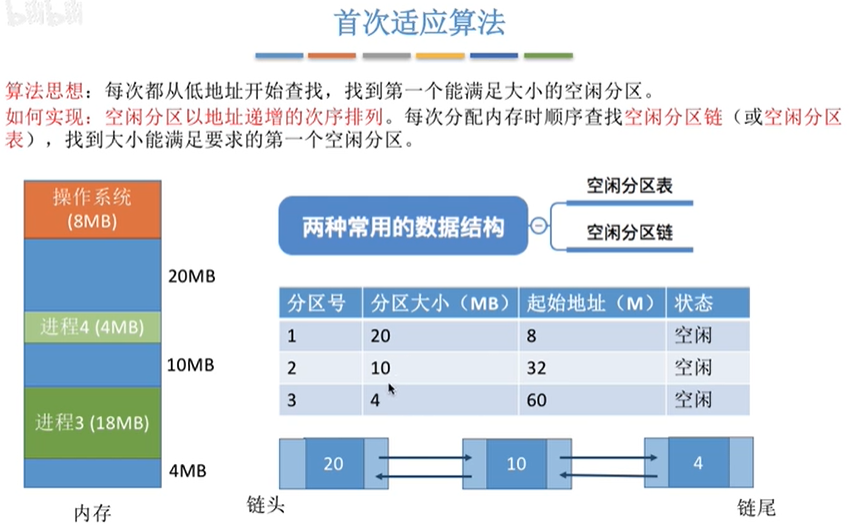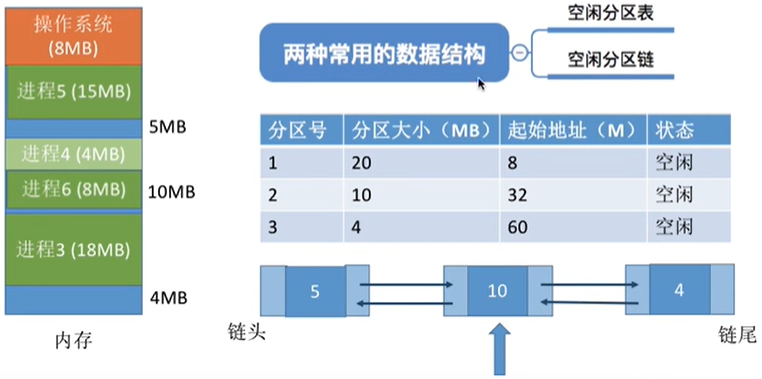2、最佳适应算法（Best Fit）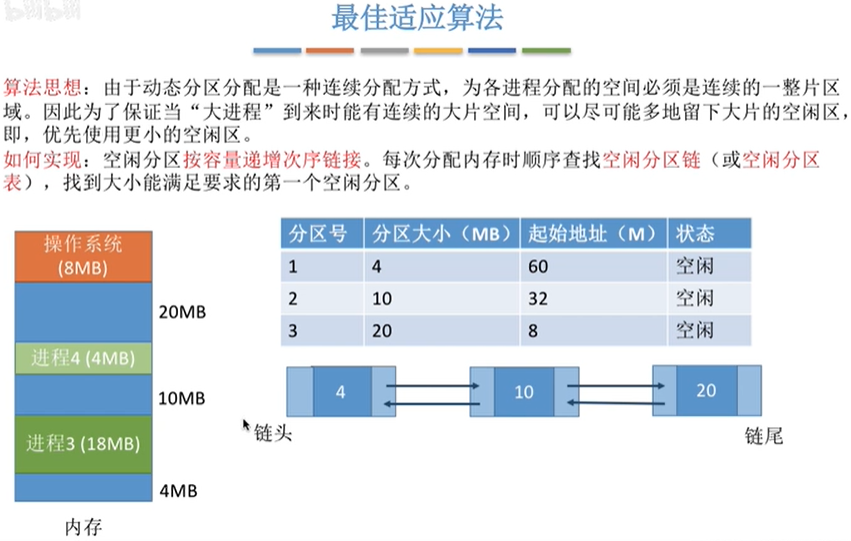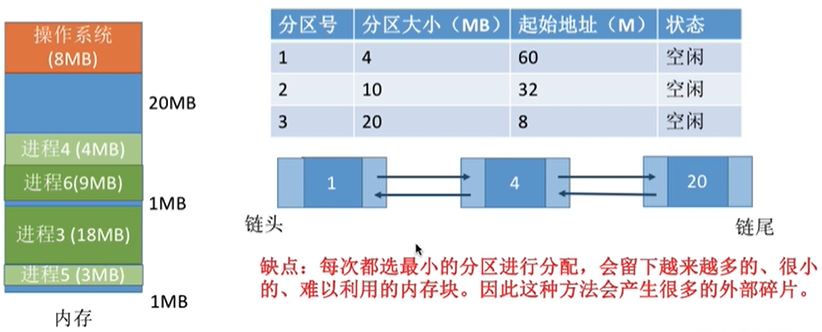3、最坏适应算法（Worst Fit）【又称“最大适应算法”（Largest Fit）】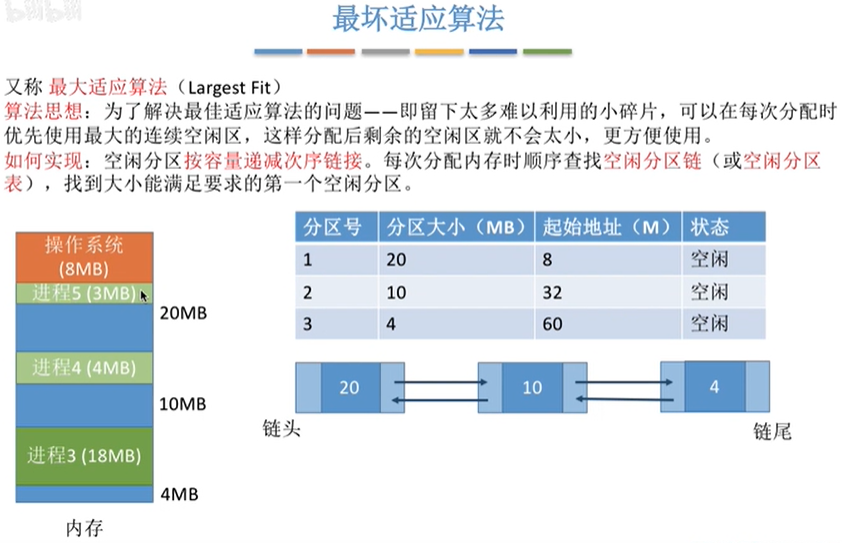空闲分区链，必须按照容量递减的次序进行排序；若不满足容量递减的次序，则重新进行排序！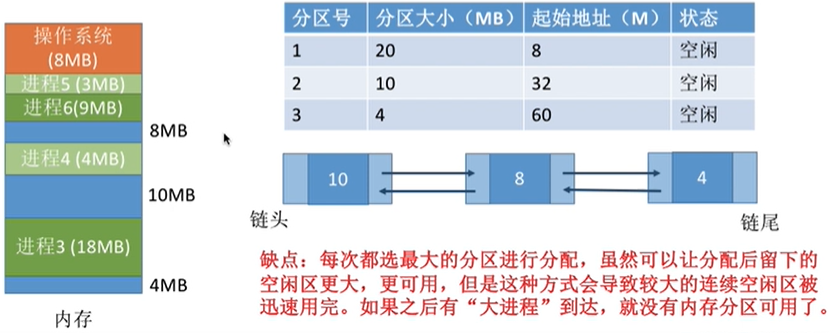4、临近适应算法（Next Fit）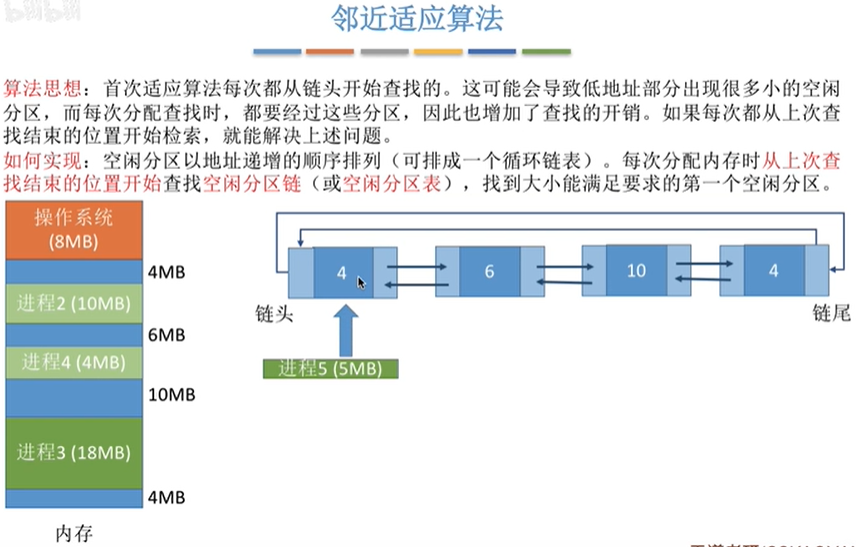空闲分区以地址递增的顺序进行排列。内存分区的大小发生了变化，并不需要对整个链表重新进行排序。算法开销小。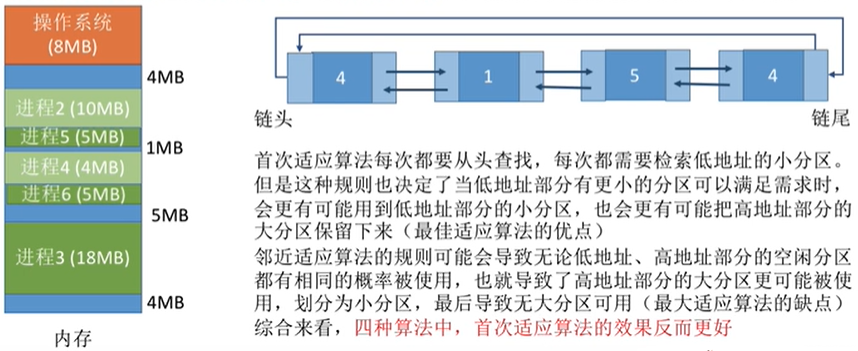算法总结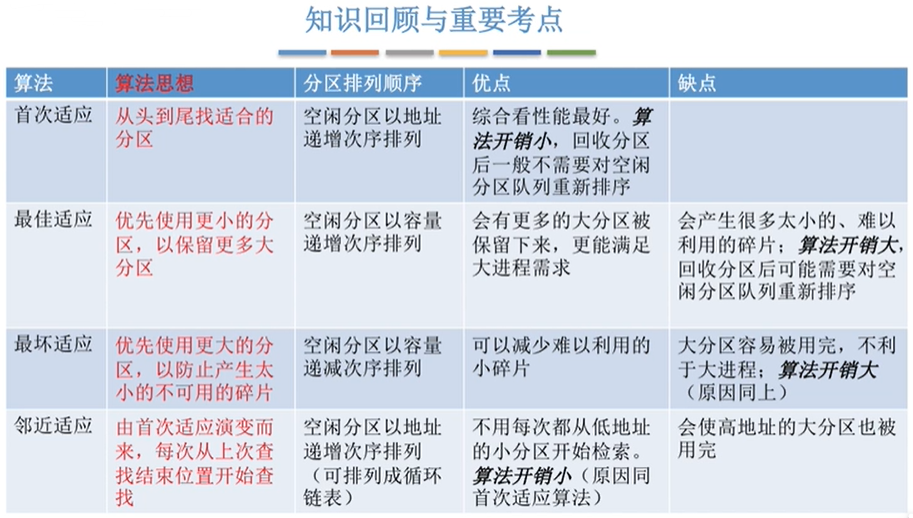算法  算法思想  分区排列顺序  优点  缺点  首次适应算法（First Fit）  从头到尾寻找合适的分区  空闲分区以地址递增次序排列  综合看，首次适应算法性能最好。算法开销小，回收分区后，一般不需要对空闲分区队列重新排序     最佳适应算法（Best Fit）  优先使用更小的分区，以保留更多的大分区  空闲分区以容量递增次序排列  会有更多的大分区被保留下来，更能满足大进程需求  会产生很多太小的、难以利用的碎片：算法开销大，回收分区后可能需要对空闲分区队列重新排序  最坏适应算法（Worst Fit） 【又称“最大适应算法”（Largest Fit）】  优先使用更大的分区，以防止产生太小的不可用碎片  空闲分区以容量递减次序排列  可以减少难以利用的小碎片  大分区容易被用完，不利于大进程：算法开销大（原因同上）  临近适应算法（Next Fit）  由首次适应算法演变而来，每次从上次查找结束的位置开始查找  空闲分区以地址递增次序排列（可排列成循环链表）  不用每次都从低地址的小分区开始检索。算法开销小（原因同首次适应算法）  会使高地址的大分区也被用完

展开全文首次适应算法 最坏适应算法 最大适应算法
• 1.首次适应算法(FF):将所有空闲分区按照地址递增的次序链接，在申请内存分配时，从链首开始查找，将满足需求的第一个空闲分区分配给作业。 void FirstFit() { cout << "***********首次适应算法***********...
动态分区分配是根据进程的实际需要，动态地址为之分配内存空间，在分配时按照一定的分配算法，从空闲分区表或空闲分区链中选出一分区分配给该作业
1.首次适应算法(FF):将所有空闲分区按照地址递增的次序链接，在申请内存分配时，从链首开始查找，将满足需求的第一个空闲分区分配给作业。  2.循环首次适应算法（NF）：将所有空闲分区按照地址递增的次序链接，在申请内存分配时，总是从上次找到的空闲分区的下一个空闲分区开始查找，将满足需求的第一个空闲分区分配给作业  3.最坏适应算法(WF)：将所有的空闲分区按照从大到小的顺序形成空闲分区链，在申请内存分配时，总是把满足需求的、最大的空闲分区分配给作业。  4.最佳适应算法(BF): 将所有空闲分区按照从小到大的顺序形成空闲分区链，在申请内存分配时，总是把满足需求的、最小的空闲分区分配给作业。
源码
#include <iostream>
#include <fstream>
#include <iomanip>
using namespace std;

#define MAXNUMBER 100
static int PartitionNum;  //内存中空闲分区的个数
static int ProcessNum; //需要分配的进程个数
static int FreePartition[MAXNUMBER];  //空闲分区对应的内存
static int ProcessNeed[MAXNUMBER];  //需要分配的进程大小

static int LeftFreePartition[MAXNUMBER];
static int LeftProcessNeed[MAXNUMBER];

static char ProcessName[MAXNUMBER];
static char NameProcessToPartition[MAXNUMBER][MAXNUMBER];

typedef struct
{
int partitionSize;
int id;
}sortNeed;

void input();
void display();
void FirstFit();
void NextFit();
void BestFit();
void WorstFit();
void selectAlgorithm(int chooceAlgorithm);
void display();

{
cout<<"请输入空闲分区数"<<endl;
cin >> PartitionNum;
cout << "请输入空闲分区大小" << endl;
for (int i = 0; i<PartitionNum; i++)
{
cin >> FreePartition[i];
}
cout<<"请输入进程个数"<<endl;
cin >> ProcessNum;
cout<<"请输入进程名称"<<endl;
for (int i = 0; i<ProcessNum; i++)
{
cin >> ProcessName[i];
}
cout<<"请输入进程需要分配大小"<<endl;
for (int i = 0; i<ProcessNum; i++)
{
cin >> ProcessNeed[i];
}
}

void input()
{
int chooseAlgorithm = 5;
do
{
cout << "请选择实现的算法：" << endl;
cout << "*****  1 - 首次适应算法     *****" << endl;
cout << "*****  2 - 循环首次适应算法 *****" << endl;
cout << "*****  3 - 最佳适应算法     *****" << endl;
cout << "*****  4 - 最坏适应算法     *****" << endl;
cout << "*****  0 - 结束             *****" << endl;
cout << "chooseAlgorithm = ";
cin >> chooseAlgorithm;
selectAlgorithm(chooseAlgorithm);
//display();
} while (chooseAlgorithm);
}

void initial()
{
for (int i = 0; i<PartitionNum; i++)
{
LeftFreePartition[i] = FreePartition[i];
for (int j = 0; j<ProcessNum; j++)
{
NameProcessToPartition[i][j] = NULL;
}
}
for (int i = 0; i<ProcessNum; i++)
{
LeftProcessNeed[i] = ProcessNeed[i];
}
}

void FirstFit()
{
cout << "***********首次适应算法***********" << endl;
initial();

int i, j;
for (i = 0; i<ProcessNum; i++)   //逐个遍历每个进程
{
for (j = 0; j<PartitionNum; j++)
{
if (LeftProcessNeed[i] <= LeftFreePartition[j] && LeftFreePartition != 0)  //当系统内存分区足够大的时候，即分配给进程资源
{
LeftFreePartition[j] -= LeftProcessNeed[i];   //扣除分配给进程的资源
LeftProcessNeed[i] = 0;  //当且仅当系统内存分区足够时才执行，即当前进程大小置0

NameProcessToPartition[i][j] = ProcessName[i];  //存储各个进程所在的分区位置

break;   //!!!很重要，一个进程分区完后，应该立即break，进行下一个进程的判断
}
}
}

display();

}

void NextFit()
{
cout << "***********循环首次适应算法***********" << endl;
initial();
int i, nextPoint = 0;
bool isWhile;
for (i = 0; i<ProcessNum; i++)
{
isWhile = true;
while (isWhile)
{
if (LeftFreePartition[nextPoint] >= LeftProcessNeed[i])
{
LeftFreePartition[nextPoint] -= LeftProcessNeed[i];
LeftProcessNeed[i] = 0;
NameProcessToPartition[i][nextPoint] = ProcessName[i];
nextPoint++;
if (nextPoint > PartitionNum - 1)
{
nextPoint = 0;  //当j遍历到分区末尾的时候，返回首位置
}
isWhile = false;
}
else
nextPoint++;
}
}
display();
}

void BestFit()
{
//思想：利用冒泡排序对分区大小进行排序，但不改变原分区的位置
//创建一个结构体，包括分区大小和所对应的id，排序过程中，每改变顺序一次，id随着改变
//关键：每次分配完一个进程的内存大小后，都要重新排序
cout << "***********最佳适应算法***********" << endl;
initial();
int i, j, temp, tempID;

sortNeed best[MAXNUMBER];
for (i = 0; i<PartitionNum; i++)
{
//初始化结构体
best[i].partitionSize = FreePartition[i];
best[i].id = i;
}

for (i = 0; i<ProcessNum; i++)
{
for (int s = PartitionNum - 1; s > 0; s--)   //冒泡排序(每次分配完一个进程后，都需要重新排序)
{
for (int t = 0; t < s; t++)
{
if (best[s].partitionSize < best[t].partitionSize)
{
temp = best[s].partitionSize;
best[s].partitionSize = best[t].partitionSize;
best[t].partitionSize = temp;

tempID = best[s].id;
best[s].id = best[t].id;
best[t].id = tempID;
}
}
}

for (j = 0; j<PartitionNum; j++)
{
if (LeftProcessNeed[i] <= best[j].partitionSize)
{
best[j].partitionSize -= LeftProcessNeed[i];
LeftProcessNeed[i] = 0;

NameProcessToPartition[i][best[j].id] = ProcessName[i];
break;
}
}
LeftFreePartition[best[j].id] = best[j].partitionSize;
}

display();
}

void WorstFit()
{
cout << "***********最坏适应算法***********" << endl;
initial();
int i, j, s, t, tempSize, tempID;
sortNeed Worst[MAXNUMBER];

for (i = 0; i<PartitionNum; i++)
{
Worst[i].partitionSize = FreePartition[i];
Worst[i].id = i;
}

for (i = 0; i<ProcessNum; i++)
{
for (s = PartitionNum - 1; s>0; s--)
{
for (t = 0; t<s; t++)
{
if (Worst[s].partitionSize > Worst[t].partitionSize)
{
tempSize = Worst[s].partitionSize;
Worst[s].partitionSize = Worst[t].partitionSize;
Worst[t].partitionSize = tempSize;

tempID = Worst[s].id;
Worst[s].id = Worst[t].id;
Worst[t].id = tempID;
}
}
}

for (j = 0; j<PartitionNum; j++)
{
if (LeftProcessNeed[i] <= Worst[j].partitionSize)
{
Worst[j].partitionSize -= LeftProcessNeed[i];
LeftProcessNeed[j] = 0;

NameProcessToPartition[i][Worst[j].id] = ProcessName[i];
break;
}
}
LeftFreePartition[Worst[j].id] = Worst[j].partitionSize;
}

display();

}

void selectAlgorithm(int chooseAlgorithm)
{
switch (chooseAlgorithm)
{
case 0:break;
case 1:FirstFit(); break;
case 2:NextFit(); break;
case 3:BestFit(); break;
case 4:WorstFit(); break;
default:cout << "请输入正确的序号：" << endl;
}
}

void display()
{
int i;
cout << "需要分配内存的进程名：" << setw(10);
for (i = 0; i<ProcessNum; i++)
{
cout << ProcessName[i] << setw(6);
}
cout << endl;
cout << "需要分配内存的进程分区大小：" << setw(4);
for (i = 0; i<ProcessNum; i++)
{
cout << ProcessNeed[i] << setw(6);
}
cout << endl;
cout << "分配结果：" << endl;

cout << "分区序号:";
for (i = 0; i<PartitionNum; i++)
{
cout << "分区" << i + 1 << " ";
}
cout << endl << "分区大小:";
for (i = 0; i<PartitionNum; i++)
{
cout << FreePartition[i] << "     ";
}
cout << endl << "剩余大小: ";
for (i = 0; i<PartitionNum; i++)
{
cout << LeftFreePartition[i] << "     ";
}
cout << endl << "分配进程情况:" << endl;
for (i = 0; i<PartitionNum; i++)
{
for (int j = 0; j<ProcessNum; j++)
{
if (NameProcessToPartition[j][i] != NULL)
{
cout << NameProcessToPartition[j][i] << ": (分区" << i + 1 << ")" << endl;
}
}
//cout<<"  ";
}
cout << endl << "********结束**********" << endl;
}

int main()
{
input();
return 0;
}

展开全文操作系统
• 用c 语言实现的最佳适应算法。 用C语言或C++语言分别实现采用首次适应算法和最佳适应算法的动态分区分配过程allocate()和回收过程reclaim()
• 分配算法　首次适应算法 最佳适应算法 循环首次适应算法 有流程图 源代码
• 分配方式可分为四类:单一连续分配、固定分区分配、动态分区分配以及动态可重定位分区分配算法四种方式，其中动态分区分配算法就是此实验的实验对象。动态分区分配又称为可变分区分配，它是根据进程的实际需要，动态...
操作系统——动态分配
写的时间早了，有些许漏洞和不足，请大家不要介意
分配方式可分为四类:单一连续分配、固定分区分配、动态分区分配以及动态可重定位分区分配算法四种方式，其中动态分区分配算法就是此实验的实验对象。动态分区分配又称为可变分区分配，它是根据进程的实际需要，动态地为之分配内存空间。在实现动态分区分配时，将涉及到分区分配中所用的数据结构、分区分配算法和分区的分配与回收操作这样三方面的问题。动态分区分配又称为可变分区分配，它是根据进程的实际需要，动态地为之分配内存空间。在实现动态分区分配时，将涉及到分区分配中所用的数据结构、分区分配算法和分区的分配与回收操作这样三方面的问题。
大体流程图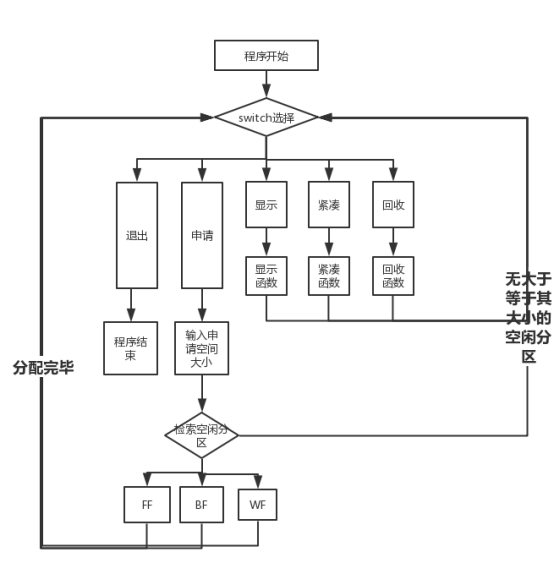算法实现原理
定义的结构体：
struct Partition
{
Partition* Back;//指向上一个分区
int Id;//分区号
int Size;//分区大小
int State;// 分区状态 0空闲 1占用
Partition* Next;//指向下一个分区
};
首次适应算法：
空闲分区以地址递增的次序排列。每次分配内存时顺序查找空闲分区链（或空闲分区表），找到大小能满足要求的第一个空闲分区。
void First_Fit(Partition* Partition_Table_Head, int Size)  //首次适应算法
{
int x = 1;
Partition* p=NULL, * q=NULL;//定义两个指针
while (p)
{
if (p->Size >= Size && p->State == 0) break;

p = p->Next;
}
//利用循环找到一个空闲的块，且其空间大小要大于等于要申请的空间
if (p->Size == Size)  p->State = 1;//若大小恰好合适，只需需改其状态即可

else
{
q = (Partition*)malloc(sizeof(Partition));
(p->Back)->Next = q;
p->Size = p->Size - Size;
q->Back = p->Back;
q->Size = Size;
q->State = 1;
q->Next = p;
p->Back = q;
}
//若大于，则新建一个块，填写其数据，并修改当前块数据，加到其之前
while (p)
{
p->Id = x;
x++;
p = p->Next;
}//x重新给所有分区编号

}
最佳适应算法：
空闲分区按容量递增次序链接。每次分配内存时顺序查找空闲分区链（或空闲分区表），找到大小能满足要求的第一个空闲分区。
void Best_Fit(Partition* Partition_Table_Head, int Size) //最佳适应算法
{
int x = 1, count = 0, Remain_Size = 0;//conut用来保证第一个找到的分区可以直接用，Remain-size用来标识最好的大小
Partition* p = nullptr;
Partition* q = nullptr;//定义两个空指针
while (p)
{

if (p->Size >= Size && p->State == 0)//找到一个空闲区，其大小大于等于要申请的大小
{
if (count == 0) //若是第一个找到的合适分区，则直接赋给q,并计算Remian_size
{
Remain_Size = p->Size - Size;
q = p;
count++;
}
else if (count != 0 && p->Size - Size < Remain_Size)若非第一个找到的合适分区，则比较Remain_size,选择小的重新赋给q
{
Remain_Size = p->Size - Size;
q = p;
}

}

p = p->Next;
}
//同理，利用循环找到“最佳”的那个空闲区
p = q;
if (p->Size == Size)  p->State = 1;

else
{
q = (Partition*)malloc(sizeof(Partition));
(p->Back)->Next = q;
p->Size = p->Size - Size;
q->Back = p->Back;
q->Size = Size;
q->State = 1;
q->Next = p;
p->Back = q;
}

while (p)
{
p->Id = x;
x++;
p = p->Next;
}
//同理，为新的分区前写信息
}
最坏适应算法：
空闲分区按容量递减次序链接。每次分配内存时顺序查找空闲分区链（或空闲分区表），找到大小能满足要求的第一个空闲分区。
void Worst_Fit(Partition* Partition_Table_Head, int Size) //最坏适应算法
{
int x = 1, count = 0, Remain_Size = 0;
Partition* p = nullptr;
Partition* q = nullptr;
while (p)
{

if (p->Size >= Size && p->State == 0)
{
if (count == 0)
{
Remain_Size = p->Size - Size;
q = p;
count++;
}
else if (count != 0 && p->Size - Size > Remain_Size)
{
Remain_Size = p->Size - Size;  //道理同最佳适应算法，只是在这块选择Remain_size大的
q = p;
}

}

p = p->Next;
}

p = q;
if (p->Size == Size)  p->State = 1;

else
{
q = (Partition*)malloc(sizeof(Partition));
(p->Back)->Next = q;
p->Size = p->Size - Size;
q->Back = p->Back;
q->Size = Size;
q->State = 1;
q->Next = p;
p->Back = q;
}

while (p)
{
p->Id = x;
x++;
p = p->Next;
}

}

回收：
对回收一个分区的四种情况，进行处理
void Recycle(Partition* Partition_Table_Head)  //回收
{

int x = 1, id;
Partition* p=NULL, * q=NULL;//定义两个空指针
cout << "请输入要回收分区的分区号 ：" << endl;
cin >> id;
while (p)
{
if (p->Id >= id) break;

p = p->Next;
}

p->State = 0;  //根据输入要回收的分区号，使p指向它，并修改其状态为空闲

check_again:	if (p->Back != NULL && (p->Back)->State == 0) //检查上一个分区是否为空，若为空，则合并
{
p->Size = p->Size + (p->Back)->Size;

if ((p->Back)->Back != NULL)	((p->Back)->Back)->Next = p;
else p->Back = NULL;

q = p->Back;
p->Back = (p->Back)->Back;
free(q);
goto check_again;  //再次检查，避免连续的空闲（虽然没可能）
}

if (p->Next != NULL && (p->Next)->State == 0) //检查下一个分区是否为空i，若为空，则合并
{
p->Size = p->Size + (p->Next)->Size;
q = p->Next;

if ((p->Next)->Next != NULL) p->Next = (p->Next)->Next;
else p->Next = NULL;
free(q);
goto check_again; //再次检查，避免连续的空闲（虽然没可能）
}

while (p)
{
p->Id = x;
x++;
p = p->Next;
}  //重新编号

}
算法效果
初始化空白分区并显示进程目录
跳过
首次适应算法、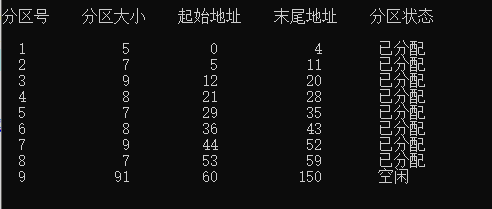回收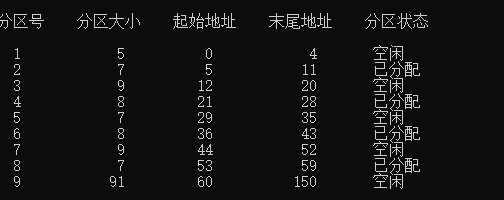回收8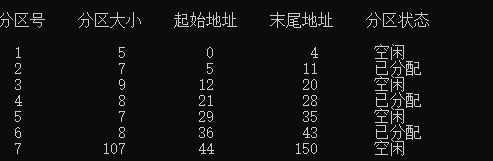最佳适应算法
申请大小为7的分区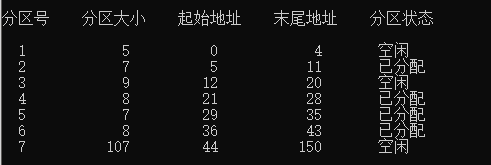最坏适应算法
申请大小为5的分区每种算法的优劣
4.1首次适应算法：
优点：高址部分的大的空闲分区得到保留，为大作业的内存分配创造了条件；
缺点：（1）每次都是优先利用低址部分的空闲分区，造成低址部分产生大量的外 部碎片
（2）每次都是从低址部分查找，使得查找空闲分区的开销增大；
4.2最佳适应算法：
优点：第一次找到的空闲分区是大小最接近待分配内存作业大小的；
缺点:产生大量难以利用的外部碎片
4.3最坏适应算法：
优点：效率高，分区查找方便；
缺点：当小作业把大空闲分区分小了，那么，大作业就找不到合适的空闲分区。

完整代码https://download.csdn.net/download/weixin_43887148/18829611
展开全文操作系统 c++ 链表
• 操作系统中利用最佳适应算法 最坏适应算法 循环首次适应算法 首次适应算法实现动态内存的分配和回收内存
• 操作系统 循环首次适应算法 首次适应算法 最佳适应算法 回收内存 分配内存设计一个可变式分区分配的存储管理方案。并模拟实现分区的分配和回收过程。 对分区的管理法可以是下面三种算法： 首次适应算法 循环首次...
• 存储管理实验（3个） 首次适应算法,循环首次适应算法,最佳适应算法
• 最佳适应算法和最坏适应算法by Evaristo Caraballo 通过Evaristo Caraballo 算法：好，坏和丑陋 (Algorithms: The Good, The Bad and The Ugly) Who has been in Free Code Camp without having the experience of...

最佳适应算法和最坏适应算法
by Evaristo Caraballo
通过Evaristo Caraballo
算法：好，坏和丑陋 (Algorithms: The Good, The Bad and The Ugly)
Who has been in Free Code Camp without having the experience of spending hours trying to solve Algorithms?
谁曾参加Free Code Camp，却没有花费大量时间尝试求解算法 ？
At Free Code Camp, we wanted to know why that was the case, and what more we could do to help you a bit.
在Free Code Camp，我们想知道为什么会这样，以及我们还能做些什么来帮助您。
We made two different analyses to have a better idea of what was going on. Data were collected between Nov 2014 to Dec 2015.
我们进行了两种不同的分析，以更好地了解正在发生的事情。 数据收集时间为2014年11月至2015年12月。
One of those analyses consisted of tracking the number of times people pasted their code to be checked by others in relevant Gitter chatrooms. We got data from the Gitter API. After some clean-up we tried to get the names of the functions of each challenge from posted messages at the Help chatroom. Although the data are not precise, it’s a good approximation of what could be happening.
这些分析之一是跟踪人们在相关的Gitter聊天室中粘贴代码以供其他人检查的次数。 我们从Gitter API获取数据。 进行一些清理后，我们尝试从帮助聊天室中发布的消息中获取每个挑战的功能名称。 尽管数据不精确，但是可以很好地近似所发生的事情。
The chart above speaks clearly on its own: algorithms like palindromes, title case, seek and destroy, longest word, reverse string, mutation or chunky monkey are those where many people ask for assistance.
上面的图表清楚地说明了自己：回文，标题大小写，查找和销毁，最长的单词，反向字符串，变异或矮胖的猴子之类的算法是许多人寻求帮助的算法。
Another analysis we made was to take the average time per page that each camper spent on each challenge, using Google Analytics data.
Again, the ugly ones are challenges like palindromes, but there are other ones that also look ugly (let’s say 1/4 of the average time per level over the same average time) specially for basic and intermediate levels, like Spinal Tap Case, Pig Latin, Search and Replace, Common Multiple, Sum All Primes, Steamroller, Friendly Date Range, Pairwise, and others.
同样，丑陋的挑战是诸如回文集之类的挑战，但是还有其他挑战看起来也很丑陋(例如，在同一平均时间内每个级别的平均时间的1/4)， 特别是对于基础水平和中级水平而言 ，例如Spinal Tap Case，Pig拉丁语，搜索和替换，公倍数，所有素数之和，Steamroller，友好日期范围，成对和其他。
Looking at the results, can we suggest the factors that are affecting the performance of campers with the algorithms?
从结果来看 ， 我们能否通过算法提出影响露营者性能的因素 ？
The most apparent reasons, given in a tentative ordering, are:
按暂定顺序给出的最明显的原因是：
Campers are finding hard to deal specially with strings, and Regex is a bad word, no matter the level! 营员发现很难对付弦 ，而正则表达式无论级别高低都是一个坏词！ There are some numeric challenges that are making camper’s life difficult, particularly those which are suitable for recursive calls. 有一些数字难题使露营者的生活变得困难，尤其是那些适合递归调用的难题。 Another common problem is dealing with nested collections of arrays/objects. 另一个常见的问题是处理数组/对象的嵌套集合 。 Difficulty with concepts and definitions is usual. For example, the concept of “symmetric difference” (with a Free Code Camp algorithm with the same name) is usually troubling because many campers don’t understand the concept properly, despite the inclusion of a widely accepted mathematical definition. 通常很难理解概念和定义 。 例如，“对称差异”的概念(使用具有相同名称的Free Code Camp算法)通常令人不安，因为尽管包含了广泛接受的数学定义，但许多营员仍无法正确理解该概念。
Similarly, looking at the last chart you may be wondering why the time per page seems not to reflect the difficulty of the problem? One explanation could be that the basic and intermediate algorithms are been taken by campers who are just learning to code or seeing JavaScript for the first time. However this is a tentative explanation and may require more analyses.
同样，查看最后一个图表，您可能想知道为什么每页时间似乎不能反映问题的难度 ？ 一种解释可能是，基本算法和中间算法是由刚开始学习编码或第一次看到JavaScript的营员采用的。 但是，这只是一个初步的解释，可能需要更多的分析。
If you are reading this and you have already suffered with some of the algorithms, you will realize that you are not alone. For those who are starting with algorithms, I would recommend the following:
如果您正在阅读本文，并且已经受了某些算法的困扰，您将意识到自己并不孤单。 对于那些开始使用算法的人，我建议以下内容：
Happy Coding!
编码愉快！
I’m also working on related visualizations at bl.ocks.org/evaristoc.
我还在bl.ocks.org/evaristoc上进行相关的可视化工作。
This analysis just scratches the surface of what we can learn from Free Code Camp’s open data. Join our Data Science chat room and help us make sense of all these data.
这种分析只是从表面上我们可以从Free Code Camp的开放数据中学到什么。 加入我们的数据科学聊天室 ，帮助我们理解所有这些数据。

最佳适应算法和最坏适应算法

展开全文• 关于首次适应算法、最佳适应算法和最差适应算法，先看一下百度百科的解释，已经说出了三者的最大区别。 首次适应算法（first-fit）： 从空闲分区表的第一个表目起查找该表，把最先能够满足要求的空闲区分配给作业...
• 文章目录前言知识总览1、首次适应算法2、最佳适应算法3、最坏适应算法4、邻近适应算法知识回顾与重要考点 前言 此篇文章是我在B站学习时所做的笔记，大部分图片都是课件老师的PPT，方便复习用。此篇文章仅供学习参考...操作系统 内存管理
• 【操作系统】分区分配算法 （首次适应算法、最佳适应算法）（C语言实现） （编码水平较菜，写博客也只是为了个人知识的总结和督促自己学习，如果有错误，希望可以指出） 1.动态分区分配算法： 为了实现动态分区分配...操作系统 c语言 链表
• 首次适应算法和最佳适应算法及内存回收，用C语言实现，测试正确。
• 这个学期学习操作系统，其实主要就是系统对于一些情况的应对算法，我们要做的就是写代码模拟这个情况，来了解操作系统是怎么解决的。 一、简介 提高内存管理的效率始终是操作系统研究的重要课题之一，虚拟存储技术是...操作系统
• //判断是否是最佳适应算法 void init(); void FF(); void alloc(MEMORY *,MEMORY *);//首次适应算法分配内存 void free(MEMORY *);//首次适应算法回收内存 void sort(MEMORY *);//对内存链进行排序 void insert...
• 最佳适应算法（Best Fit）：该算法总是把既能满足要求，又是最小的空闲分区分配给作业。为了加速查找，该算法要求将所有的空闲区按其大小排序后，以递增顺序形成一个空白链。这样每次找到的第一个满足要求的空闲区，...操作系统
• 用C语言(不会C这里就用JS实现)分别实现采用首次适应算法和最佳适应算法的动态分区分配过程alloc( )和回收过程free( )。其中，空闲分区通过空闲分区链来管理：在进行内存分配时，系统优先使用空闲区低端的空间。 (2)...操作系统 JS 首次适应算法
• #include<stdio.h> #define getpch(type) (type)malloc(sizeof(type) struct LNode { int size; int start; int end; struct LNode *next; struct LNode *front; }*L; /*L 为头指针 */ typedef struct LNode LN;...
• 湖南师范大学 信息科学与工程学院 操作系统课程实验报告 ...参照教材P137-P140的内容，编写一个C程序，用char *malloc（unsigned size）函数向系统申请一次内存空间（如size=1000，单位为字节），用循环首次适应算法c++ 操作系统
• 实验题目 设计和实现关于内存管理的内存布局初始化及内存申请分配、内存回收等基本功能操作函数，尝试对用256MB的内存空间进行动态...比较次数少于最佳适应算法（Best Fit），内存利用率低于最佳适应算法（Best Fit）。...